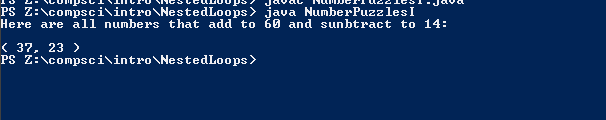# Assignment #115 NumberPuzzlesI

## Code

```        /// Name: Lacey Reese
/// Period: 5
/// Program Name:  NumberPuzzlesI
/// File Name:  NumberPuzzlesI.java
/// Date Finished 4/29/2016

public class NumberPuzzlesI
{

public static void main( String[] args )
{

System.out.println( "Here are all numbers that add to 60 and sunbtract to 14:" );
System.out.println();

for( int i=0; i<=60; i++ ) {

for( int n=0; n<=60; n++ ) {
int c = n+i;
int d = n-i;

if ( c == 60 ) {
if ( d == 14 ) {
System.out.println( "( " + n + ", " + i + " )" );
}
}
}
}

}
}

Picture of the output```